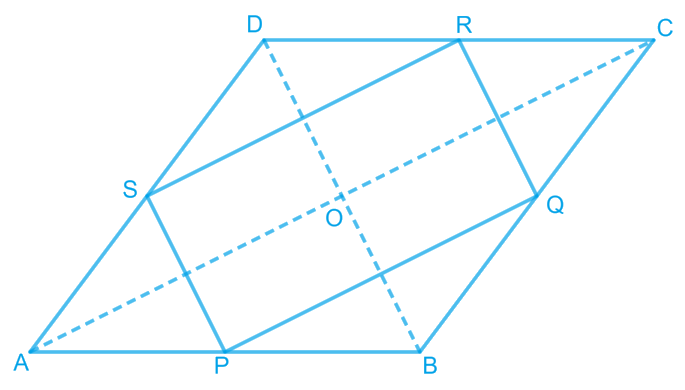# Ex.8.2 Q2 Quadrilaterals Solution - NCERT Maths Class 9

Go back to  'Ex.8.2'

## Question

$$ABCD$$ is a rhombus and $$P$$, $$Q$$, $$R$$ and $$S$$ are the mid-points of the sides $$AB$$, $$BC$$, $$CD$$ and $$DA$$ respectively. Show that the quadrilateral $$PQRS$$ is a rectangle.

Video Solution
Ex 8.2 | Question 2

## Text Solution

What is known?

$$ABCD$$ is a rhombus and $$P, Q, R$$ and $$S$$ are the mid-points of the sides $$AB, BC, CD$$and $$DA$$ respectively.

What is unknown?

How we can show that quadrilateral $$PQRS$$ is a rectangle.

Reasoning:

In a triangle, the line segment joining the mid-points of any two sides of the triangle is parallel to the third side and is half of it. Also if one pair of opposite sides of quadrilateral is parallel and equal to each other then it is a parallelogram. By showing one of the angle in parallelogram as $$90$$ degree then we can say it is rectangle.

Steps:In $$\rm \Delta ABC,$$ $$P$$ and $$Q$$ are the mid-points of sides $$AB$$ and $$BC$$ respectively.

\therefore \begin{align} \rm PQ \parallel AC \; and \; PQ =\frac{1}{2} \text{AC} \end{align}  (Using mid-point theorem).....(1)

In $$\Delta \text{ADC,}$$

$$R$$ and $$S$$ are the mid-points of $$CD$$ and $$AD$$ respectively.

\therefore \begin{align} RS \parallel AC \; and \; RS=\frac{1}{2} \text{AC } \end{align} (Using mid-point theorem) ....(2)

From Equations (1) and (2), we obtain

\begin{align} PQ \parallel RS \; and \; PQ= RS \end{align}

Since in quadrilateral $$PQRS$$, one pair of opposite sides is equal and parallel to each other, it is a parallelogram.

Let the diagonals of rhombus $$ABCD$$ intersect each other at point $$O$$.

In quadrilateral $$OMQN$$,

\begin{align} MQ \parallel ON \; \; ( \because PQ \parallel AC) \end{align}

\begin{align} QN \parallel OM \; \; ( \because QR \parallel BD) \end{align}

Therefore, $$OMQN$$ is a parallelogram.

\begin{align} \therefore \angle MQN = \angle NOM \end{align}

\begin{align} \therefore \angle PQR = \angle NOM \end{align}

However,\begin{align} \angle NOM = 90^\circ\end{align} (Diagonals of a rhombus are perpendicular to each other)

\begin{align} \therefore \angle PQR = 90^\circ\end{align}

Clearly, $$PQRS$$ is a parallelogram having one of its interior angles as $$90^\circ$$ .

Hence, $$PQRS$$ is a rectangle.

Video Solution